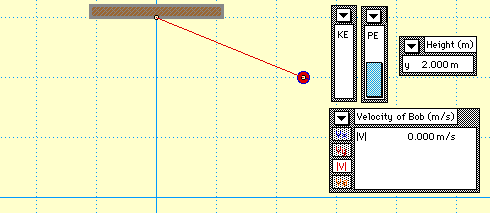## Energy Transformation for a Pendulum

The motion of a pendulum is a classic example of mechanical energy conservation. A pendulum consists of a mass (known as a bob) attached by a string to a pivot point. As the pendulum moves it sweeps out a circular arc, moving back and forth in a periodic fashion. Neglecting air resistance (which would indeed be small for an aerodynamically shaped bob), there are only two forces acting upon the pendulum bob. One force is gravity. The force of gravity acts in a downward direction and does work upon the pendulum bob. However, gravity is an internal force (or conservative force) and thus does not serve to change the total amount of mechanical energy of the bob. The other force acting upon the bob is the force of tension. Tension is an external force and if it did do work upon the pendulum bob it would indeed serve to change the total mechanical energy of the bob. However, the force of tension does not do work since it always acts in a direction perpendicular to the motion of the bob. At all points in the trajectory of the pendulum bob, the angle between the force of tension and its direction of motion is 90 degrees. Thus, the force of tension does not do work upon the bob.

Since there are no external forces doing work, the total mechanical energy of the pendulum bob is conserved. The conservation of mechanical energy is demonstrated in the animation below. Observe the KE and PE bars of the bar chart; their sum is a constant value.Observe that the falling motion of the bob is accompanied by an increase in speed. As the bob loses height and PE, it gains speed and KE; yet the total of the two forms of mechanical energy is conserved.

To test you understanding of the conservation principle, use the heights and the speeds given in the table below to fill in the remaining cells at the various locations in a 0.200-kg bob's trajectory. Use the pull down menus to check your work.

(Note: mass = 0.200 kg)

h
(m)
Speed
(m/s)
PE
(J)
KE
(J)
TME
(J)
2.000 0.0 m/s
1.490
3.920 J
1.128
0.897
0.357

For more information on physical descriptions of motion, visit The Physics Classroom Tutorial. Detailed information is available there on the following topics:

Potential Energy

Kinetic Energy

Mechanical Energy

Internal vs. External Forces

Work

Work-Energy Theorem

Work-Energy Bar Charts